# Introduction to Oce

#### 2019-06-16

Abstract. The oce package makes it easy to read, summarize and plot data from a variety of Oceanographic instruments, isolating the researcher from the quirky data formats that are common in this field. It also provides functions for working with basic seawater properties such as the equation of state, and with derived quantities such as the buoyancy frequency. Although simple enough to be used in a teaching context, oce is powerful enough for a research setting. These things are illustrated here in a general context; see also the vignettes on using data-quality flags and processing data from acoustic Doppler profilers.

# 1 Introduction

Oceanographers must deal with measurements made by a wide variety of instruments, a task that is complicated by a tendency of instrument manufacturers to invent new data formats. Although manufacturers often provide software for scanning data files and producing overview plots, this software is of limited use to researchers who work with several instrument types at the same time, and who need to move beyond engineering plots to scientific plots and statistical analysis.

# 2 Architecture of oce objects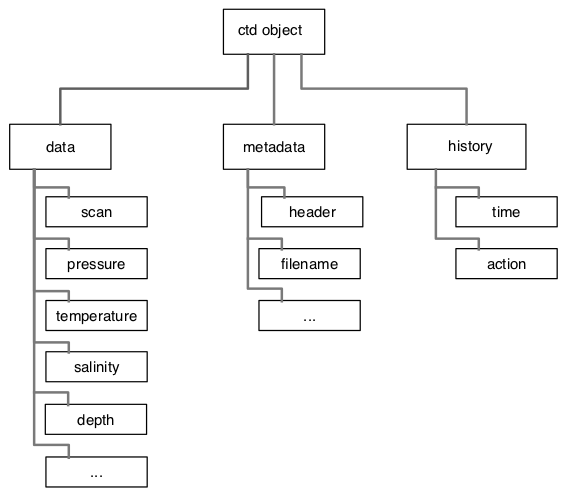Figure 1. Basic layout of a CTD object. All oce objects contain slots named data, metadata, and processingLog, with the contents depending on the type of data.

The need to scan diverse data files was one motivation for the creation of oce, but an equal goal was to make it easy to work with the data once they are in the system. This was accomplished partly by the provision of specialized and generic (overloaded) functions to work with the data, and partly by providing accessor methods that make it convenient to reach inside the data objects (see next section).

As illustrated in Figure 1, each oce object contains three slots:

• data, a list containing the actual data, e.g., for a CTD object, this will contain pressure, temperature, etc.
• metadata, a list containing information about the data, such as units, data-quality flags, sampling locations, etc.
• processingLog, a list that documents how the object was created and possibly changed thereafter.

# 3 Accessing data in oce objects

For detailed analysis, users may want to access data within oce objects. While it is possible to descend through the object using the “slot” and “list” notation (e.g. d@data$salinity yields the salinity within an oce object named d), this approach is not recommended. It is better to use the [[ notation, which derives from the generic “Extract” method for accessing parts of an object. A general introduction is provided by ?[[,oce-method and details are provided for individual object classes with e.g. ?[[,ctd-method for the ctd class. The notation is very simple. For example, suppose that d is an object that stores salinity in either its data slot or metadata slot. Then, S <- d[['salinity']] will extract the salinity. The [[ method first looks in the metadata slot, but if the item is not found there, it proceeds to look in the data slot. This two-step scheme is helpful because it frees the user from having to know where data are stored, e.g. in a ctd object, latitude might be stored in the metadata (for a conventional CTD cast) or in the data slot (for the slantwise sampling of a glider). In addition to accessing data within an object, the [[ notation also permits the extraction of derived information. There are two reasons for this. 1. It can give valuable performance enhancements in some cases, e.g. the data in the landsat class are stored in two byte-level arrays, which yields a marked improvement over the 8-byte arrays that R generally uses on a 64-bit machine. The [[ assembles these byte-scale chunks into a conventional R numerical matrix, which can be quite convenient for users who wish to operate on the data without learning how to assemble the bytes. 2. It provides a uniformity of notation that can be helpful to users. In oce, objects derived from data files tend to hold just the information in those files, not derived information. For example, For example, CTD datasets often provide in-situ temperature but not potential temperature (since the latter is not measured). The in-situ temperature is found with e.g. d[["temperature"]], and so it seems natural to write d[["theta"]] to get the potential temperature. If this is done, then the ctd version of [[ first looks in the data slot, and returns theta if it is found there, as may sometimes be the case (depending on the choice of the person who created the dataset). However, if it is not found, then [[ calls swTheta() to calculate the value, and returns the result. Finally, it is also possible to extract the entirety of either the metadata or data slot, e.g. data <- d[['data']] yields the full data slot, which is a list with elements that can be accessed in the conventional way, e.g. for a ctd object, data$temperature

retrieves the temperature. For obvious reasons, the method of derived-quantity access (e.g. the theta of the example above) will not work.

# 4 Modifying data in oce objects

There are several schemes for modifying the data within oce objects. By analogy with the [[ notation of the previous section, e.g. the following

data(ctd)
ctd[["temperatureAboveFreezing"]] <- ctd[["temperature"]] - swTFreeze(ctd)

will store the excess over freezing temperature into the ctd object.

Further information on this notation is provided by

?"[[<-,oce-method"

The above works only within the data slot. To store within the metadata slot, consider using e.g.

ctd[["metadata"]]$scientist <- "Dalhousie Oceanography 4120/5120 Class of 2003" sets the “scientist”. For archival work, it is important to store reasons for changing things in an object. Two functions are provided for this purpose: oceSetData and oceSetMetadata. For example, a better way to change the scientist might be to write ctd <- oceSetMetadata(ctd, name="scientist", value="Dalhousie Oceanography 4120/5120 Class of 2003", note="give credit where it's due") and a better way to store temperatureAboveFreezing would be ctd <- oceSetData(ctd, name="temperatureAboveFreezing", value=ctd[["temperature"]] - swTFreeze(ctd), unit=list(unit=expression(degree*C), scale="ITS-90"), originalName="-", note="add temperatureAboveFreezing, for ice-related calculations") which illustrates that this notation, as opposed to the [[<- notation, permits the specification of a unit and an originalName, both of which, together with the note, are displayed by summary(ctd). # 5 Oce functions The uniformity of the various oce objects helps users build skill in examining and modifying objects. Fine-scale control is provided throughout oce, but the best way to learn is to start with simplest tools and their defaults. For example, the following will read a CTD file named "station1.cnv", summarize the contents, and plot an overview of the data, with profiles, a TS diagram, and a map (Figure 2). library(oce) d <- read.oce("station1.cnv") summary(d) plot(d) The reader should stop now and try this on a file of their own. The pattern will work with a fairly wide variety of file types, because read.oce() examines the file name and contents to try to discover what it is. For an example, if read.oce() is given the name of a file created by an Acoustic Doppler Profiler, it will return an object inheriting from class "adp", so the summary() and plot() calls will be tailored to that type, e.g. the graph will show images of time-distance variation in each of the measured velocity components. Notes on oce function names. 1. As just illustrated, the general function to read a dataset ends in .oce, and the name is a signal that the returned object is of class oce. Depending on the file contents, d will also have an additional class, e.g. if the file contains CTD data, then the object would inherit from two classes, oce and ctd, with the second being used to tailor the graphics by passing control to the ctd variant of the generic plot function (use help("plot,ctd-method") to learn more). 2. Generally, oce functions employ a “camel case” naming convention, in which a function that is described by several words is named by stringing the words together, capitalizing the second and subsequent words. For example, ctdAddColumn() takes a ctd object, and returns a new ctd object that matches the original except for the added column (and an added entry in the processingLog to indicate the addition). 3. Function names begin with oce in cases where a more natural name would be in conflict with a function in the base R system or a package commonly used by Oceanographers. For example, oceContour() is a function that provides an alternative to contour() in the graphics package. # 6 Calculation of seawater properties The oce package provides many functions for dealing with seawater properties. Perhaps the most used is swRho(S,T,p), which computes seawater density $$\rho=\rho(S, T, p)$$, where $$S$$ is salinity, $$T$$ is in-situ temperature in $$^\circ$$C (on the ITS-90 scale), and $$p$$ is seawater pressure, i.e. the excess over atmospheric pressure, in dbar. (This and similar functions starts with the letters sw to designate that they relate to seawater properties.) The result is a number in the order of $$1000$$kg/m$$^3$$. For many purposes, Oceanographers prefer to use the density anomaly $$\sigma=\rho-1000$$kg/m$$^3$$, provided with swSigma(salinity,temperature,pressure), or its adiabatic cousin $$\sigma_\theta$$, provided with swSigmaTheta(). Most of the functions can use either the UNESCO or GSW (TEOS-10) formulation of seawater properties, with the choice set by an argument called eos. It should be noted that oce uses the gsw package for GSW calculations. Caution: the results obtained with eos="gsw" in oce functions may differ from the results obtained when using the gsw functions directly, due to unit conventions. For example, swCSTp(..., eos="gsw") reports conductivity ratio for consistency with the UNESCO formulation, however the underlying gsw function gsw::gsw_C_from_SP() reports conductivity in mS/cm. A partial list of seawater functions is as follows: • swAlpha() for thermal expansion coefficient $$\alpha=-\rho_0^{-1}\partial\rho/\partial T$$ • swAlphaOverBeta() for $$\alpha/\beta$$ • swBeta() for haline compression coefficient $$\beta=\rho_0^{-1}\partial\rho/\partial S$$ • swConductivity() for conductivity from $$S$$, $$T$$ and $$p$$ • swDepth() for depth from $$p$$ and latitude • swDynamicHeight() for dynamic height • swLapseRate() for adiabatic lapse rate • swN2() for buoyancy frequency • swRho() for density $$\rho$$ from $$S$$, $$T$$ and $$p$$ • swSCTp() for salinity from conductivity, temperature and pressure • swSTrho() for salinity from temperature and density • swSigma() for $$\rho-1000$$,kg/m$$^3$$ • swSigmaT() for $$\sigma$$ with $$p$$ set to zero and temperature unaltered • swSigmaTheta() for$$\sigma$$ with $$p$$ set to zero and temperature altered adiabatically • swSoundSpeed() for speed of sound • swSpecificHeat() for specific heat • swSpice() for a quantity used in double-diffusive research • swTFreeze() for freezing temperature • swTSrho() for temperature from salinity and density • swTheta() for potential temperature $$\theta$$ • swViscosity() for viscosity Details and examples are provided in the documentation of these functions. The following exercise may be of help to readers who prefer to learn by doing. (Answers are provided at the end of this document.) Exercise 1. a. What is the density of a seawater parcel at pressure 100 dbar, with salinity 34 PSU and temperature 10$$^\circ$$C? b. What temperature would the parcel have if raised adiabatically to the surface? c. What density would it have if raised adiabatically to the surface? d. What density would it have if lowered about 100m, increasing the pressure to 200 dbar? e. Draw a blank $$T$$-$$S$$ diagram with $$S$$ from 30 to 40 PSU and $$T$$ from -2 to 20$$^\circ$$C. # 7 CTD data ## 7.1 Example with pre-trimmed data Many of the object types supported by oce come with built-in data. For an example, data(ctd) yields a CTD profile that has been trimmed to just the downcast portion of the sampling. (See the next section to learn how to do this trimming.) A summary and plot (Figure 2) are created as follows. library(oce) data(ctd) summary(ctd) #> CTD Summary #> ----------- #> #> * Instrument: SBE 25 #> * Temp. serial no.: 1140 #> * Cond. serial no.: 832 #> * File: "/Users/kelley/git/oce/create_data/ctd/ctd.cnv" #> * Original file: c:\seasoft3\basin\bed0302.hex #> * Start time: 2003-10-15 15:38:38 #> * Sample interval: 1 s #> * Cruise: Halifax Harbour #> * Vessel: Divcom3 #> * Station: Stn 2 #> * Location: 44.684N 63.644W #> * Data #> #> Min. Mean Max. Dim. OriginalName #> scan 130 220 310 181 scan #> timeS [s] 129 219 309 181 timeS #> pressure [dbar] 1.48 22.885 44.141 181 pr #> depth [m] 1.468 22.698 43.778 181 depS #> temperature [°C, IPTS-68] 2.919 7.5063 14.237 181 t068 #> salinity [PSS-78] 29.916 31.219 31.498 181 sal00 #> flag 0 0 0 181 flag #> #> * Processing Log #> - 2018-11-14 16:03:47 UTC: create 'ctd' object #> - 2018-11-14 16:03:47 UTC: read.ctd.sbe(file = file, debug = 10, processingLog = processingLog) #> - 2018-11-14 16:03:47 UTC: oce.edit(x = ctd, item = "startTime", value = as.POSIXct(gsub("1903", "2003", format(ctd[["startTime"]])), tz = "UTC") + 4 * 3600, reason = "file had year=1903, instead of 2003", person = "Dan Kelley") plot(ctd)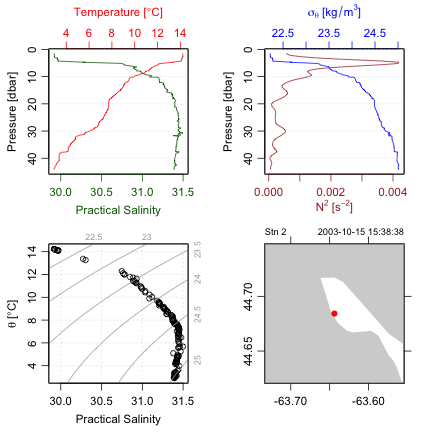Figure 2. An overview of a ctd dataset. Accessing the data within this ctd object can be done directly, e.g. ctd@data$pressure holds the pressure record, but it is usually better to use an accessor function that is provided with oce. This function is named [[, and it takes a character string as an argument, e.g. ctd[["pressure"]] yields the pressure column. The accessor notation is preferable to direct access because it is simpler for the user. For example, several oce objects store the data in single-byte or two-byte chunks, to match the raw format used by the instruments, and the accessor function takes care of translating these values to what are sometimes called “science” units.

Exercise 2. Plot a profile of $$\sigma_\theta$$ and $$N^2$$, for the data in the pycnocline. (Hint: use subset().)

## 7.2 Example with raw data

Practicing Oceanographers may be wondering how the CTD cast used in the preceding section was trimmed of equilibration-phase and upcast-phase data. Spurious data from these phases must be trimmed as a first step in processing. For example, consider the following code.

data(ctdRaw)
plotScan(ctdRaw)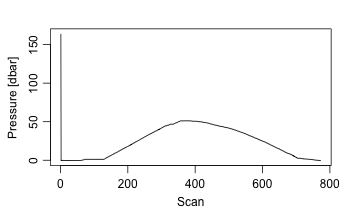Figure 3. Scanwise plot of the ctdRaw sample data set. Note the spike at the start, the equilibration phase before the downcast, and the spurious freshening signal near the start of the upcast.

This produces a two-panel plot (Figure 3) of the data as a time-series, revealing not just the desired downcast, but also an earlier equilibration phase and a later upcast. The x-axis in Figure 3 is the scan number, which is a convenient index for extraction of the downcast portion of the profile by an essentially manual method, e.g. proceeding with a sequence of commands such as

plotScan(ctdTrim(ctdRaw, "range",
parameters=list(item="scan", from=140, to=250)))
plotScan(ctdTrim(ctdRaw, "range",
parameters=list(item="scan", from=150, to=250)))

This method of making decisions based on plotted information is probably the most robust method of trimming data. However, for quick work, users may be satisfied with the results of automatic downcast detection, e.g.

ctdTrimmed <- ctdTrim(ctdRaw)

It should be noted that ctdTrim() inserts entries into the object’s log file, so that the details of how the trimming was done are recorded together with the data.

Once the profile has been trimmed, one may wish to use ctd.decimate() to smooth the data and interpolate the smoothed results to uniformly-spaced pressure values.

Taking these things together, a quick visual examination of a CTD file takes just one line of code:

plot(ctdDecimate(ctdTrim(read.ctd("stn123.cnv"))))

## 7.3 Example with WOCE archive data

The package has a harder time scanning the headers of data files in the WOCE archive format than it does in the Seabird format illustrated in the previous examples. This is mainly because front-line researchers tend to work in the Seabird format, and partly because the WOCE format is odd. For example, the first line of a WOCE file is of the form CTD,20060609WHPOSIODAM (or BOTTLE,...). Scanning the item to the left of the comma is not difficult (although there are variants to the two shown, e.g. CTDO sometimes occurs). The part to the right of the comma is more difficult. The first part is a date (yyyymmdd) so that is no problem. But then things start to get tricky. In the example provided, this text contains the division of the institute (WHPO), the institute itself (SIO), and initial of the investigator (DAM). The problem is that no dividers separate these items, and that there seem to be no standards for the item lengths. Rather than spend a great deal of time coding special cases (e.g. scanning to see if the string WHOI occurs in the header line), the approach taken with oce is to ignore such issues relating to quirky headers, on the assumption that users can scan human-written headers with high skill.

Quite commonly, CTD profiles taken during a cruise are collected together in a sequence of files in a given directory. For a real-world example, one might visit the website mentioned in the code provided below, download and expand the zip file, enter the directory thus formed, and run the code to get an overall TS plot for all the CTD stations of this cruise. (Caution: the link seems to change from time to time.)

library(oce)
# http://cchdo.ucsd.edu/data/7971/ar18_58JH19941029_ct1.zip
files <- system("ls *.csv", intern=TRUE)
for (i in 1:length(files)) {
if (i == 1) {
plotTS(x, Slim=c(31, 35.5), Tlim=c(-2, 10), type='o')
} else {
points(x[["salinity"]], x[["potential temperature"]])
lines(x[["salinity"]], x[["potential temperature"]])
}
}

The [[ notation is explained in Section 3, but this example conveys the gist, that it permits accessing data, or derived data, from within an object.

In the above, lines connect the points within a given profile. This can be a useful method for a quick scan looking for outliers. Another is to colour-code the profiles, although this gets confusing with large datasets, in which case the method of the following exercise might be useful.

Exercise 3. (advanced) Make a multi-file plot summarizing the TS relationship, with each file showing the overall relationship in gray and the individual profile in black.

# 8 Section plots

The commands

data(section)
plot(section, which=c(1, 2, 3, 99))

will plot a summary diagram containing sections of $$T$$, $$S$$, and $$\sigma_\theta$$, along with a chart indicating station locations. In addition to such overview diagrams, the section variant of the generic plot function can also create individual plots of individual properties (use help("plot,section-method") to learn more).

Some section datasets are supplied in a pre-gridded format, but it is also common to have different pressure levels at each station. For such cases, sectionGrid() may be used, e.g. the following produces Figure 4. The ship was travelling westward from the Mediterranean towards North America, taking 124 stations in total; the stationId value selects the last few stations of the section, during which the ship headed toward the northwest, crossing isobaths (and perhaps, the Gulf Stream) at nearly right angles.

library(oce)
data(section)
GS <- subset(section, 102 <= stationId & stationId <= 124)
GSg <- sectionGrid(GS, p=seq(0, 1600, 25))
plot(GSg, which=c(1,99), map.xlim=c(-85,-(64+13/60)))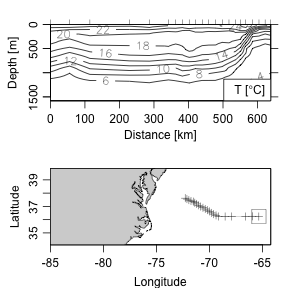Figure 4. Portion of the CTD section designated A03, showing the Gulf Sream region. The square on the cruise track corresponds to zero distance on the section.

Exercise 4. Draw a $$T$$-$$S$$ diagram for the section data, using black symbols to the east of 30W and gray symbols to the west, thus highlighting Mediterranean-derived waters. Use handleFlags() (see using data-quality flags) to discard questionable data, and use the accessor function [[.

Exercise 5. Plot dynamic height and geostrophic velocity across the Gulf Stream. (Hint: use the swDynamicHeight() function.)

# 9 Maps

There are several ways to handle map projections in R and other systems. Oce uses the rgdal package to calculate the details of map projections. A wide group of functions is provided for plotting maps. The first step is to call mapPlot() to construct the map, after which points can be added with mapPoints(), lines with mapLines(), etc. For example, the following plots a world coastline in the Winkel Tripel projection, with the cruise track for the section dataset in red.

library(oce)
data(coastlineWorld)
par(mar=rep(0, 4))
mapPlot(coastlineWorld, projection="+proj=robin", col="lightgray")
data(section)
lon <- section[["longitude", "byStation"]]
lat <- section[["latitude", "byStation"]]
mapLines(lon, lat, col='red', cex=0.5)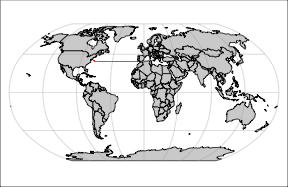Figure 5. World in Robinson projection, with the section profile sites indicated.

There are far too many projections to illustrate here. See http://dankelley.github.io/r/2015/04/03/oce-proj.html for a blog item that provides examples of the available projections. Note that some have a problem with spurious horizontal lines. This can result from coastline segments that cross the edge of the plotting area, and getting rid of this is tricky enough to be the heart of the longest-lived bug in the oce issue list, i.e. https://github.com/dankelley/oce/issues/388. In some instances the function coastlineCut() can help, but it is provisional and subject to change.

# 10 Sea-level data

## 10.1 Time-domain analysis

The following code graphs a built-in dataset of sea-level time series (Figure 9). The top panel provides an overview of the entire data set. The second panel is narrowed to the most recent month, which should reveal spring-neap cycles if the tide is mixed. The third panel is a log spectrum, with a few tidal constituents indicated. The section variant of the generic plot function provides other possibilities for plotting, including a cumulative spectrum that can be quite informative (use help("plot,sealevel-method") to learn more).

library(oce)
data(sealevel)
plot(sealevel)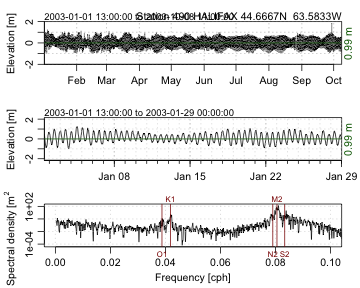Figure 9. Sea-level timeseries measured in 2003 in Halifax Harbour.

Exercise 6. Illustrate Halifax sea-level variations during Hurricane Juan, near the end of September, 2003.

## 10.2 Tidal-harmonic analysis

A preliminary version of tidal analysis is provided by the tidem function provided in this version of the package, but readers are cautioned that the results are certain to change in a future version. (The problems involve phase and the inference of satellite nodes.)

Exercise 7. Plot the de-tided Halifax sea level during Autumn 2003, to see whether Hurricane Juan is visible. (Hint: use predict with the results from tidem.)

# 11 Acoustic Doppler Profiler data

The following commands produce Figure 10, showing one velocity component of currents measured in the St Lawrence Estuary Internal Wave Experiment. This plot type is just one of many provided by the adp variant of the generic plot function (see ?"plot,adp-method"). See the adp vignette for much more on acoustic Doppler profiler data.

library(oce)
lines(adp[['time']], adp[['pressure']], lwd=2)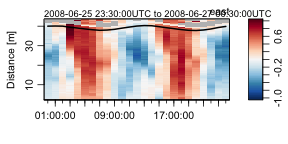Figure 10. Measurements made with a bottom-mounted ADP in the St Lawrence Estuary. The line near the surface indicates pressure measured by the ADP.

# 12 Handling data-quality flags

Archives of CTD/bottle and Argo drifter measurements commonly supply data-quality flags that provide an indication of the trust to be put in individual data points. Oce has a flexible scheme for dealing with such flags, and also for inserting flags into these or any data type; see the using data-quality flags vignette for more..

# 13 Working in non-English languages

Many of the oce plotting functions produce axis labels that can be displayed in languages other than English. At the time of writing, French, German, Spanish, and Mandarin are supported in at least a rudimentary way. Setting the language can be done at the general system level, or within R, as indicated below (results not shown).

library(oce)
Sys.setenv(LANGUAGE="fr")
data(ctd)
plot(ctd)

Most of the translated items were found by online dictionaries, and so they may be incorrect for oceanographic usage. Readers can help out in the translation effort, if they have knowledge of how nautical words such as Pitch and Roll and technical terms such as Absolute Salinity and Potential Temperature should be written in various languages.

# 14 Extending oce

## 14.1 Initializing oce objects

Oce handles a wide variety of data types, creating sub-classes the inherit from the base class that is named oce. However, even if a dataset does not fit within these sub-classes, it is still possible to use some of the features of oce, such as (FILL IN … accessors, plotting, summaries ??).

For example, suppose that there is a dataset that contains two variables, mm and ss, that have been measured at times t. Suppose that the data are recorded at a fixed location. To illustrate how to deal with such data, we first create some fake data:

lon <- -60
lat <- -30
t <- seq(as.POSIXct("2017-08-30"), as.POSIXct("2017-09-01"), by="15 min")
tsec <- as.numeric(t)
u <- sin(tsec * 2 * pi / (3600 * 12.4206))
v <- 0.5 * cos(tsec * 2 * pi / (3600 * 12.4206))

These may be inserted into an oce object as follows. First, create the object.

o <- new("oce")

This contains slots without data:

str(o)
#> Formal class 'oce' [package "oce"] with 3 slots
#>   ..@ metadata     :List of 2
#>   .. ..$units: list() #> .. ..$ flags: list()
#>   ..@ data         : list()
#>   ..@ processingLog:List of 2
#>   .. ..$time : POSIXct[1:1], format: "2019-06-16 14:44:31" #> .. ..$ value: chr "Create oce object"

As noted previously, it’s possible to insert t, u, and v directly into the data slot, but it’s better to use oceSetData to do that, because it can set up units, and it leaves a trail in the processing log.

o <- oceSetData(o, "t", t, list(unit=expression(s), scale=""))
o <- oceSetData(o, "u", u, list(unit=expression(m/s), scale=""))
o <- oceSetData(o, "v", v, list(unit=expression(m/s), scale=""))

Astute readers will realize that these data are akin to tidal velocities, and so might agree with the author that lon and lat belong in the metadata slot, not the data slot.

o <- oceSetMetadata(o, "longitude", lon)
o <- oceSetMetadata(o, "latitude", lat)

At this stage, o is a full-fledged oce object. It may be summarized with

summary(o)
#> * Data
#>
#>             Min.       Mean       Max.       Dim. OriginalName
#>     t [s]   2017-08-30 2017-08-31 2017-09-01 193  -
#>     u [m/s] -0.99982   -0.027415  1          193  -
#>     v [m/s] -0.49996   -0.0048211 0.49998    193  -
#>
#> * Processing Log
#>     - 2019-06-16 11:44:31 UTC: Create oce object
#>     - 2019-06-16 11:45:35 UTC: oceSetData(object = o, name = "t", value = t, unit = list(unit = expression(s),     scale = ""))
#>     - 2019-06-16 11:45:35 UTC: oceSetData(object = o, name = "u", value = u, unit = list(unit = expression(m/s),     scale = ""))
#>     - 2019-06-16 11:45:35 UTC: oceSetData(object = o, name = "v", value = v, unit = list(unit = expression(m/s),     scale = ""))
#>     - 2019-06-16 11:45:35 UTC: oceSetMetadata(object = o, name = "longitude", value = lon)
#>     - 2019-06-16 11:45:35 UTC: oceSetMetadata(object = o, name = "latitude", value = lat)

and individual data components may be extracted with the [[ notation

o[["latitude"]]                        # from metadata
#>  -30
#>  0.9961884 0.9772306 0.9426639 0.8930403 0.8291526 0.7520211
#>  -0.04361357 -0.10608997 -0.16687184 -0.22498833 -0.27951117 -0.32956949

A crude plot can be done with

plot(o)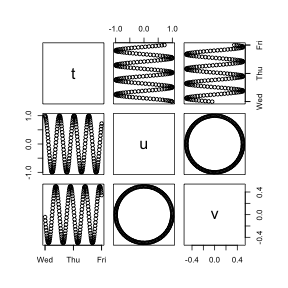Figure 11. Crude plot of constructed oce object.

Note that the plot uses the pairs function to show how all elements of the data slot vary with each other. To customize the plot, a new object class should be created, as in the next section.

## 14.2 Creating new oce object classes

Let’s call this new object class uv. With this, inventing a new object sub-class is as simple as invoking

setClass("uv", contains="oce")

For most objects, it makes sense to define three specialized methods: one to create new objects (called "initialize"), one to plot them ("plot"), and one to summarize them ("summary").

The initialization can be handled with

setMethod(f="initialize",
signature="uv",
definition=function(.Object, time, u, v, longitude, latitude) {
if (missing(time)) stop("must provide 'time'")
## repeat the above also for u, v, longitude, and latitude
.Object@metadata$units$u <- list(unit=expression(m/s), scale="")
.Object@metadata$units$v <- list(unit=expression(m/s), scale="")
.Object@metadata$longitude <- longitude .Object@metadata$latitude <- latitude
.Object@data$time <- time .Object@data$u <- u
.Object@data$v <- v .Object@processingLog$time <- presentTime()
.Object@processingLog$value <- "create 'uv' object" return(.Object) }) This is fairly arcane, but simply copying and pasting this will be enough to get the reader started. Let’s try it: oo <- new("uv", t, u, v, lon, lat) Next, customize summary for this new class: setMethod(f="summary", signature="uv", definition=function(object, ...) { cat("uv Summary\n-----------\n\n", ...) cat(paste("* Location: ", sprintf("%.5f N", object@metadata$latitude), ", ",
sprintf("%.5f E", object@metadata$longitude), "\n", sep='')) callNextMethod() }) The callNextMethod will summarize the entries in the data slot adequately for this case. Let’s try it: summary(oo) #> uv Summary #> ----------- #> #> * Location: -30.00000 N, -60.00000 E #> * Time ranges from 2017-08-30 to 2017-09-01 with 193 samples and mean increment 15 min #> * Data #> #> Min. Mean Max. Dim. OriginalName #> u [m/s] -0.99982 -0.027415 1 193 - #> v [m/s] -0.49996 -0.0048211 0.49998 193 - #> #> * Processing Log #> - 2019-06-16 14:45:36 UTC: create 'uv' object #> NULL Finally, let’s make a custom method for plot. This is usually the most complicated part of handling any new object class, because there are usually many ways to look at data. (Some oce objects have over a dozen specialized plot styles.) Let’s suppose there are only two desired plot styles: one showing timeseries of u and v in a two panel plot, and the other showing how u and v covary. setMethod(f="plot", signature=signature("uv"), definition=function(x, which=1, ...) { if (which == 1) { oce.plot.ts(x[["time"]], x[["u"]], ylab="u [m/s]", ...) } else if (which == 2) { oce.plot.ts(x[["time"]], x[["v"]], ylab="v [m/s]", ...) } else if (which == 3) { plot(x[["u"]], x[["v"]], xlab="u [m/s]", ylab="v [m/s]", ...) } }) An now, we can try it out par(mfrow=c(2,1)) plot(oo, which=1) plot(oo, which=2)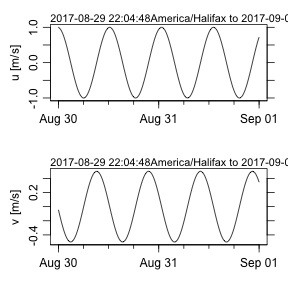Figure 12. Time-series u(t) plot of newly-constructed uv object. plot(oo, which=3, asp=1)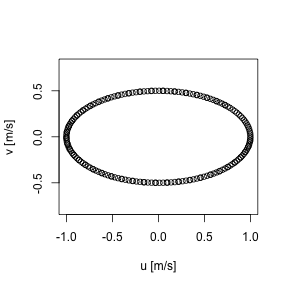Figure 13. Hodograph plot of newly-constructed uv object. # 15 The future of oce The present version of oce can only handle data types that the authors have been using in their research. New data types will be added as the need arises in that work, but the authors would also be happy to add other data types that are likely to prove useful to the Oceanographic community. (The data types need not be restricted to Physical Oceanography, but the authors will need some help in dealing with other types of data, given their research focus.) Two principles will guide the addition of data types and functions: (a) the need, as perceived by the authors or by other contributors and (b) the ease with which the additions can be made. One might call this development-by-triage, by analogy to the scheme used in Emergency Rooms to organize medical effort efficiently. # 16 Development website The site http://github.com/dankelley/oce provides a window on the development that goes on between the CRAN releases of the package. Readers are requested to visit the site to report bugs, to suggest new features, or just to see how oce development is coming along. Note that the development branch is used by the authors in their work, and is updated so frequently that it must be considered unstable, at least in those spots being changed on a given day. Official CRAN releases derive from the master branch, and are done when the code is considered to be of reasonable stability and quality. This is all in a common pattern for open-source software. # 17 Answers to exercises Exercise 1. Seawater properties. In the UNESCO system we may write library(oce) swRho(34, 10, 100, eos="unesco") #>  1026.624 swTheta(34, 10, 100, eos="unesco") #>  9.988599 swRho(34, swTheta(34, 10, 100, eos="unesco"), 0, eos="unesco") #>  1026.173 swRho(34, swTheta(34, 10, 100, 200, eos="unesco"), 200, eos="unesco") #>  1027.074 plotTS(as.ctd(c(30,40),c(-2,20),rep(0,2)), eos="unesco", grid=TRUE, col="white") and in the Gibbs SeaWater system, we use eos="gws" and must supply longitude and latitude arguments to the sw*() calls and also to the as.ctd() call. Exercise 2. Plot a profile of $$\sigma_\theta$$ and $$N^2$$, for the data in the pycnocline. (Hint: use subset().) Although one may argue as to the limits of the pycnocline, for illustration let us say it is in 5 dbar to 12 dbar range. One way to do this is library(oce) data(ctd) pycnocline <- ctdTrim(ctd, "range", parameters=list(item="pressure", from=5, to=12)) plotProfile(pycnocline, which="density+N2") Another is library(oce) data(ctd) pycnocline <- subset(ctd, 5 <= pressure & pressure <= 12) plotProfile(pycnocline, which="density+N2") Exercise 3. Make a multi-file plot summarizing the TS relationship, with each file showing the overall relationship in gray and the individual profile in black. (This is an advanced exercise requiring some R skills.) The code provided below creates 91 PNG files, with names ar18_01.png, ar18_02.png, etc. Loading these in a view that permits quick paging through this file list is an easy way to spot suspicious data, since each plot has the station number at the top. (Users trying this example should bear in mind that this is a fairly large dataset, so the processing will take up to a minute.) library(oce) # http://cchdo.ucsd.edu/data/7971/ar18_58JH19941029_ct1.zip # setwd("~/Downloads/ar18_58JH19941029_ct1") files <- system("ls *.csv", intern=TRUE) n <- length(files) ctds <- vector("list", n) # to hold the CTD objects station <- vector("list", n) for (i in 1:n) { ctds[[i]] <- read.ctd(files[i]) station[[i]] <- ctds[[i]][["station"]] } S <- unlist(lapply(1:n, function(i) ctds[[i]][["salinity"]])) T <- unlist(lapply(1:n, function(i) ctds[[i]][["temperature"]])) p <- unlist(lapply(1:n, function(i) ctds[[i]][["pressure"]])) overall <- as.ctd(S, T, p) png("ar18_%02d.png") for (i in 1:n) { plotTS(overall, col='gray') lines(ctds[[i]][["salinity"]], ctds[[i]][["potential temperature"]]) mtext(station[i], side=3, line=0) } dev.off() Exercise 4. Draw a $$T$$-$$S$$ diagram for the section data, using black symbols to the east of 30W and gray symbols to the west, thus highlighting Mediterranean-derived waters. Use handleFlags() (see using data-quality flags) to discard questionable data, and use the accessor function [[. We will use the Gibbs SeaWater system, so as.ctd() needs location information. library(oce) data(section) s <- handleFlags(section, flags=list(c(1, 3:9))) ctd <- as.ctd(s[["salinity"]], s[["temperature"]], s[["pressure"]], longitude=s[["longitude"]], latitude=s[["latitude"]]) col <- ifelse(s[["longitude"]] > -30, "black", "gray") plotTS(ctd, col=col, eos="gsw") Exercise 5. Plot dynamic height and geostrophic velocity across the Gulf Stream. (Hint: use the swDynamicHeight function.) (Try ?swDynamicHeight for hints on smoothing.) library(oce) data(section) GS <- subset(section, 102 <= stationId & stationId <= 124) dh <- swDynamicHeight(GS) #> Warning in regularize.values(x, y, ties, missing(ties)): collapsing to #> unique 'x' values par(mfrow=c(2,1), mar=c(3, 3, 1, 1), mgp=c(2, 0.7, 0)) plot(dh$distance, dh$height, type="l", xlab="", ylab="Dyn. Height [m]") grid() # 1e3 metres per kilometre latMean <- mean(GS[["latitude"]]) f <- coriolis(latMean) g <- gravity(latMean) v <- diff(dh$height)/diff(dh$distance) * g / f / 1e3 plot(dh$distance[-1], v, type="l", xlab="Distance [km]", ylab="Velocity [m/s]")
grid()
abline(h=0, col='red')

Exercise 6. Halifax sea-level during Hurricane Juan, near the end of September, 2003.

A web search will tell you that Hurricane Juan hit about midnight, 2003-sep-28. The first author can verify that the strongest winds occurred a bit after midnight – that was the time he moved to a room without windows, in fear of flying glass.

library(oce)
data(sealevel)
# Focus on 2003-Sep-28 to 29th, the time when Hurricane Juan caused flooding
plot(sealevel,which=1,xlim=as.POSIXct(c("2003-09-24","2003-10-05"), tz="UTC"))
abline(v=as.POSIXct("2003-09-29 04:00:00", tz="UTC"), col="red")
mtext("Juan", at=as.POSIXct("2003-09-29 04:00:00", tz="UTC"), col="red")

Exercise 7. Plot the de-tided Halifax sea level during Autumn 2003, to see whether Hurricane Juan is visible. (Hint: use predict with the results from tidem.)

library(oce)
data(sealevel)
m <- tidem(sealevel)
oce.plot.ts(sealevel[['time']], sealevel[['elevation']] - predict(m),
ylab="Detided sealevel [m]",
xlim=c(as.POSIXct("2003-09-20"), as.POSIXct("2003-10-08")))

The spike reveals a surge of about 1.5m, on the 29th of September, 2003.

Exercise 8. Manipulating flags.

library(oce)
d[["flags"]]$temperature <- flag1 d[["flags"]]$conductivity <- flag2
d[["flags"]]\$oxygen <- flag3